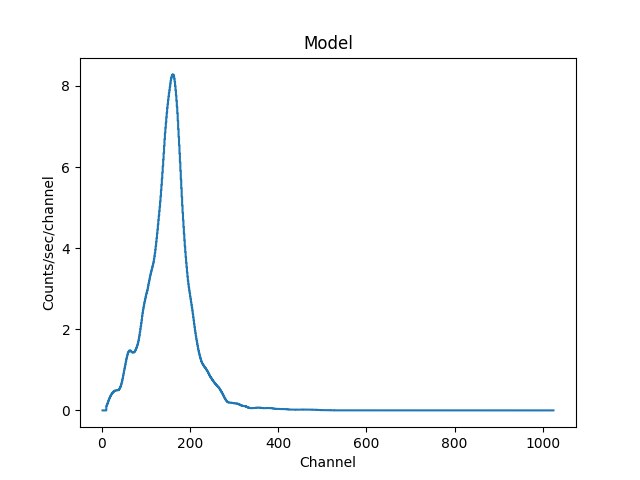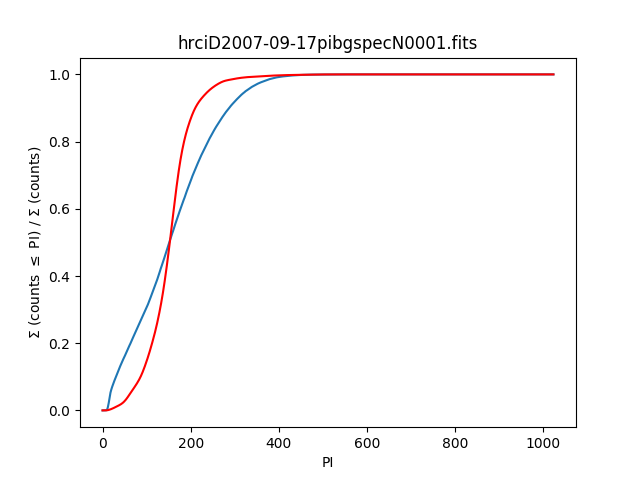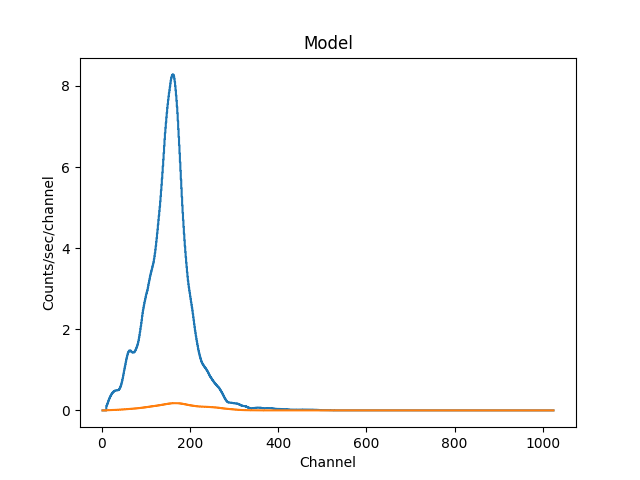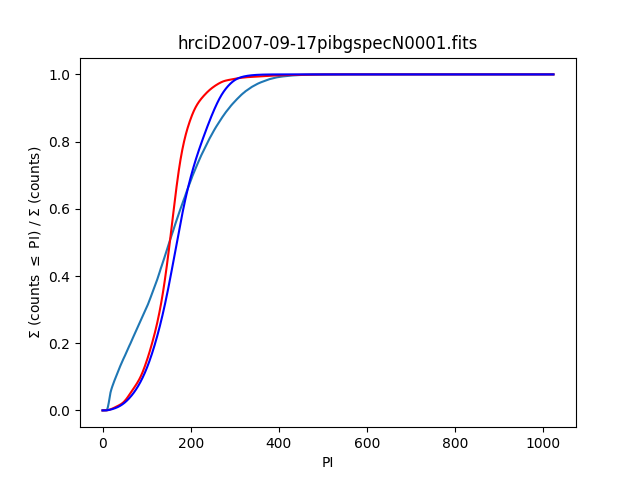The HRC-I Background Spectra FilesCIAO 4.11 Science Threads

Overview

Synopsis:

The HRC calibration team has released a set of background spectra from the HRC-I. These spectra describe the particle background of the detector and vary slowly with time which means that they can be used to improve the signal-to-noise of HRC-I imaging data. This can significantly improve signal-to-noise for extended sources and may be useful for point source analysis (e.g. fields with many point sources).

Purpose:

The intent of this thread is to apply a PI (instrumental energy channel) filter to an HRC-I imaging event file to increase the signal-to-noise of the resulting images by removing channel ranges in which the particle background dominates. Calculating the cumulative source and background PI distributions allow us to estimate the fraction of source and background counts removed by a given PI filter.

To create an HRC-I background event file tailored to a specific observation for imaging or spatial analyses, follow the HRC-I Background Event Files thread instead.

Last Update: 2 Apr 2019 - Updated to use matplotlib for plotting.

Get Started

Download the sample data: 9700 (HRC-I, Cas A)

The HRC background spectra files are included in the main CALDB download file. In contrast to the background event files, there is no additional tarfile to download.

Obtain the Response Files: ARF and RMF

This analysis requires an ARF and RMF. This section of the thread provides the necessary commands; refer to the ahelp files for asphist and mkarf for details.

For the HRC-I, a single RMF file distributed in the CALDB is used (rather than an observation specific one as for ACIS). We make a soft link to the file for easier access:

Compute the cumulative background distribution

The background spectrum is used to estimate the reduction in the background signal that the PI filtering will achieve.

unix% sherpa

>>> d = get_data()
>>> pi = d.channel-1.0
>>> bgcumul = np.cumsum(d.counts)
>>> bgcdf = bgcumul * 1.0 / bgcumul[-1]

>>> import matplotlib.pylab as plt
>>> plt.figure(1)
>>> plt.plot(pi, bgcdf, marker="None")
>>> plt.xlabel("PI")
>>> plt.ylabel(r"$\Sigma$ (counts $\leq$ PI) / $\Sigma$ (counts)")
>>> plt.title(d.name.split("/")[-1])
>>> plt.show()

Figure 1: Cumulative background distributionFigure 1: Cumulative background distribution

Note that the calculation for bgcdf assumes that d.counts is >= 0, which should be safe here.

Compute the cumulative distributions for model spectrum

We assume that the source spectrum is unknown, so in order to select the PI range we use a soft and a hard spectrum to determine suitable lower and upper limits. For this thread we use an absorbed power law in both cases, varying the absorption and slope to maximize the relative flux at low or high energies, although other approaches are possible (in particular using a model similar to that of the source).

Set up an ARF and RMF

We use the background model which we have already loaded to define the channel grid, and add an ARF and RMF to it.

>>> set_analysis("channel")

The set_analysis call is to ensure that the following modeling is done in PI channels rather than energy or wavelength units.

Set up the "soft" model

>>> set_source(xswabs.abs1*powlaw1d.pl)
>>> abs1.NH = 0.001
>>> pl.gamma = 2.5

>>> plt.figure(2)
>>> plot_model(overplot=False)
>>> plt.show()

Figure 2: Plot of the soft modelFigure 2: Plot of the soft model

The add_window call is used so that the original plot is not deleted by the call to plot_model.

Calculate the cumulative distribution

>>> scumul = np.cumsum(get_model_plot().y)
>>> scdf = scumul / max(scumul)

>>> plt.figure(1)
>>> plt.plot(pi,scdf, marker="None", color="red")
>>> plt.show()

Figure 3: Cumulative distribution with the soft modelFigure 3: Cumulative distribution with the soft model

Here we add the cumulative distribution for the soft model to the original plot, so that it can be compared to that of the background spectrum.

Set up the "hard" model

>>> abs1.nh = 10
>>> pl.gamma = 1

>>> plt.figure(2)
>>> plot_model(overplot=True)
>>> plt.show()

Figure 4: Plot of the hard modelCalculate the cumulative distribution

>>> hcumul = np.cumsum(get_model_plot().y)
>>> hcdf = hcumul / max(hcumul)

>>> plt.figure(1)
>>> plt.plot(pi,hcdf, marker="None", color="blue")
>>> plt.show()

Figure 5: Cumulative distribution with the hard modelCalculate the PI range

Various strategies could be employed to optimize the PI range. Ideally, we seek to maximize background reduction while minimizing loss of source events. In practice, the trade-off requires testing many different PI ranges and adopting one that suits best. One strategy is to require a certain amount of reduction in background, compute the appropriate PI range, and then estimate the magnitude of source event loss for a specific source model. Alternately, one could set forth an acceptable amount of source loss (sensible ranges are 1 to 10 per cent), compute the appropriate PI range, and then check how much of an improvement results in the background.

This thread follows the latter strategy. We will use a conservative strategy, and look to lose only 5% of source events, split equally over the low and high PI ranges. If xfrac is the fraction of events to exclude and is set to 5%, then we have

>>> xfrac = 0.05
>>> pimin = np.interp(xfrac/2, scdf, pi)
>>> pimax = np.interp(1-xfrac/2, hcdf, pi)
>>> lo = int(pimin)
>>> hi = int(pimax) + 1

>>> print ("PI range: %d to %d" % (lo,hi))
PI range: 47 to 293

Here we chose to round the minimum limit down and the maximum limit up; routines in the Python math module, such as floor and ceil can be used to implement different rounding strategies.

The lo to hi range can then be used to filter the level=2 event file when creating images.

Calculating the background reduction

The PI range can be viewed by saying:

>>> plt.axvline(lo, color="green")
>>> plt.axvline(hi, color="green")
>>> plt.show()

Figure 6: PI range overlaid on the modelsFigure 6: PI range overlaid on the models

and the fraction of the background that is excluded by these limits is calculated by:

>>> blo = np.interp(lo, pi, bgcdf)
>>> bhi = 1.0 - np.interp(hi, pi, bgcdf)
>>> bfrac = bhi + blo

>>> print("Fraction of background excluded is %g" % bfrac)
Fraction of background excluded is 0.242981

Filtering on the range PI=48:293 will reduce the background by ~25% while only losing ~5% of x-ray events.

Apply the PI Filter

The PI range 48:293 is applied to the file in a Data Model filter:

unix% punlearn dmcopy
unix% dmcopy "hrcf09700N003_evt2.fits[pi=48:293]" hrcf09700_evt2_pi_flt.fits

A background annulus is defined around the source to check the number of "good" events in the filtered and unfiltered files:

unix% cat bkg.reg
# Region file format: CIAO version 1.0
annulus(16368.483,16336.472,2731.4111,4097.1167)

unix% dmstat "hrcf09700N003_evt2.fits[sky=region(bkg.reg)][cols pi]" ver=0
unix% pget dmstat out_good
54713

unix% dmstat "hrcf09700_evt2_pi_flt.fits[sky=region(bkg.reg)][cols pi]" ver=0
unix% pget dmstat out_good
42567

The percent change between the files is ~22% [(54713-42567)/54713 = 22], which is close to the 24% reduction we expected.

Scripting It

The file hrci.shp performs the Sherpa commands used in this thread. One could loop over different values of xfrac to find the optimal S/N improvement.

History

 10 Jun 2010 new for CIAO 4.2/CALDB 4.3.0 11 Jan 2011 reviewed for CIAO 4.3: no changes 04 Apr 2011 updated for 04 Apr scripts package release: hrc_bkgrnd_lookup script prints the version at verbose > 0. 20 Jul 2011 required software updates are listed in Synopsis 11 Jan 2012 reviewed for CIAO 4.4 and CALDB 4.4.7: the 2010 HRC-I background PI spectrum file was remade with the new gain map (hrciD2010-09-25pibgspecN0001.fits), and the 2011 file has been added (hrciD2011-09-19pibgspecN0001.fits); added Scripting It section 03 Dec 2012 Review for CIAO 4.5; file version name changes 03 Dec 2013 Review for CIAO 4.6; no changes. 14 Apr 2014 Minor edits to text to clarify when/how to use this thread. Updated list of CALDB files. 18 Dec 2014 Reviewed for CIAO 4.7; no changes. 02 Apr 2019 Updated to use matplotlib for plotting.